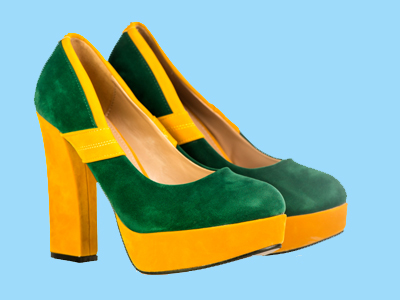Someone wearing a trainer on one foot and a stiletto on the other exerts the most pressure on the ground with the stiletto.

# Pressure 03

## Test yourself on comparisons of pressure. Is suction a pull force or a pushing force?

### Scroll down to play the quiz

This is our third KS3 Science quiz on pressure. For a given force, if it is acting on a smaller surface area, it will create a bigger pressure. Try cutting a fruit with the wide flat side of a knife. Now try the thin side - it will cut smoothly. The flat side has a greater surface area, therefore less pressure as the force is more spread out. Some of the questions that you will be asked about pressure will be about making comparisons between different objects, like in the example above. These test if you understand how pressure works. Other questions will test your knowledge of how to work out pressure, they will require you to do some calculations.

When people talk about suction, they imagine that it is a pull force. It is in fact a pushing force created by a difference in pressure. A good example is a vacuum cleaner. It creates an area of low pressure inside itself. Outside of the cleaner, the air pressure is normal but it is higher than inside. If you really understand pressure, you will realise that this creates an unbalanced force where the inside of the vacuum cleaner meets the outside world. The force created pushes dust and other objects into the vacuum cleaner.

1.
The dimensions of a ski are 8 cm x 150 cm. What is the weight of a skier standing on 2 skis to produce a pressure of 2,500 Pa?
500 N
600 N
900 N
2,400 N
Area of ski = 0.08 x 1.50 = 0.12 m2 x 2 (for 2 skis), force = pressure x area = 2,500 x 0.24 = 600 N
2.
The bottom of a box has dimensions 2 m by 3 m. What is the weight of the box to produce a pressure of 20 Pa?
4 N
26 N
100 N
120 N
Area = 2 x 3 = 6 m2, force = 20 x 6 = 120 N
3.
A four legged table exerts a pressure of 8,000 Pa on the ground. Each leg has an area of 0.01 m2. What is the weight of the table?
32 N
80 N
320 N
320 kg
Force = pressure x area = 8,000 x (4 x 0.01) = 320 N
4.
What force is needed on an area of 5 m2 to produce a pressure of 25 Pa?
5 N
20 N
30 N
125 N
Force = pressure x area = 25 x 5 = 125 N
5.
The bottom of a box has an area of 5 m2. What is the weight of the box to produce a pressure of 20 Pa?
4 N
100 N
4 kg
100 kg
Force = 20 x 5 = 100 N. Remember weight is a force so the two kilogram answers cannot possibly be correct as kilograms are the units of mass, not force
6.
What has to happen to the force exerted if the area doubles but the pressure is unchanged?
The force must decrease
The force must double
The force must increase
The force must stay the same
There is a direct positive correlation between force and area if the pressure remains the same
7.
A person at a fancy dress party is wearing a trainer on one foot and a shoe with a stiletto heel on the other! Which exerts the most pressure on the ground?
Both the same
Stiletto heel
They don't exert a pressure
Trainer
The force (person's weight) is the same on both feet but a stiletto heel has a smaller area. This is why stiletto heels can damage wooden floors
8.
Box A has a weight of 20 N. It is put on top of box B. These two boxes exert a pressure of 60 Pa. The area box B covers is 3 m2. Find weight of box B.
60 N
80 N
160 N
180 N
Force = 60 x 3 = 180 N, box A = 20 N so box B = 180-20 N
9.
What has to happen to the force exerted over a fixed area if the pressure doubles?
The force must decrease
The force must double
The force must increase
The force must stay the same
There is a direct positive correlation between force and pressure if the area remains the same
10.
What force is needed on an area of 4 m2 to produce a pressure of 16 Pa?
0.25 N
4 N
64 N
64 Kg
Force = pressure x area = 16 x 4 = 64 N. By the way, did you realise that you can immediately rule out the fourth option because Kg is incorrect, it should be kg (no capital letter)